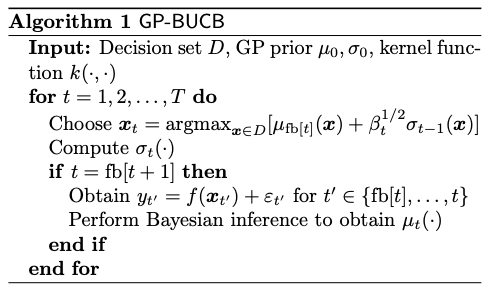## 高斯过程回归(GPR)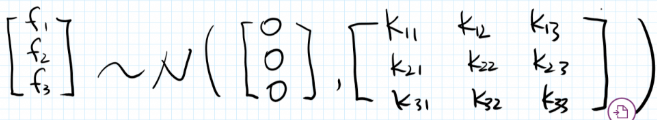• 协方差

$$k(x_1, x_2) = E[(x_1 - E(x_1))*(x_2 - E(x_2)]$$

• 相关系数

$$k(x_1, x_2) = \frac{E[(x_1 - E(x_1))*(x_2 - E(x_2)]}{\sigma_{x_1}\sigma_{x_2}}$$

• RBF kernel

$$k(x_1, x_2) = \exp(-\frac{||x_1 - x_2||_2^2}{2\sigma^2})$$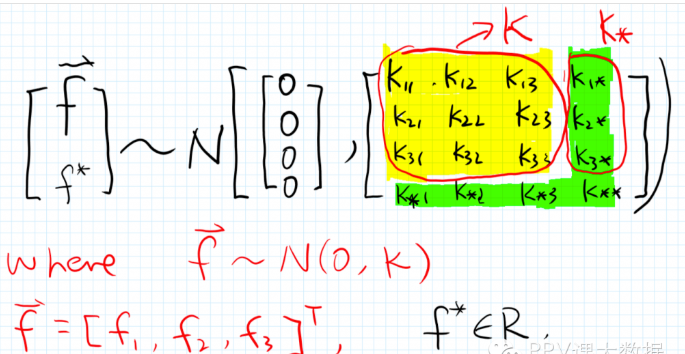$$K_* = [k(x_*, x_1), k(x_*, x_2), k(x_*, x*_*3)]^T$$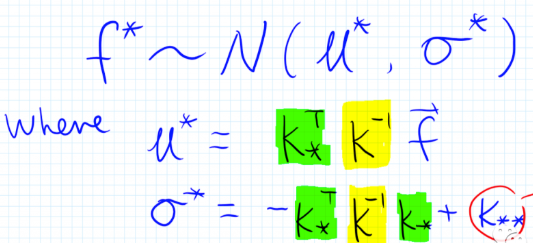$$\log p(y|x,\theta) = -\frac{1}{2}\log|K| - \frac{1}{2} (y-\mu)^TK^{-1}(y-\mu)-\frac{n}{2}\log(2\pi)$$

sklearn 中提供的 GPR 的实验，具体的使用方法及实现可参考 GaussianProcessRegressor

Gaussian Process Optimization in the Bandit Setting: No Regret and Experimental Design 中最早提出了一种应用在GPR 中的 UCB 方法, 也称为 GP-UCB，其方法的流程如下图所示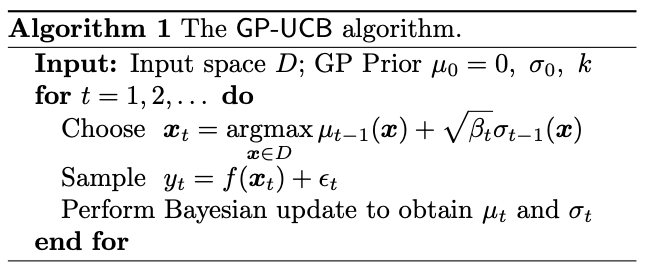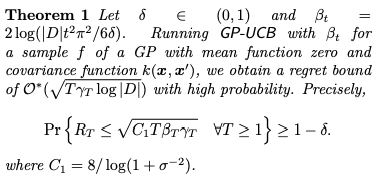GP-UCB 方法每次只能选择一个 $x$ 进行试验，因此 Parallelizing Exploration–Exploitation Tradeoffs with Gaussian Process Bandit Optimization 中对GP-UCB 进行了简单的改进，每次能够选择多个 $x$ 并行进行试验，其算法流程如下